# Test: Circles- 2

## 30 Questions MCQ Test Mathematics For JEE | Test: Circles- 2

Description
Attempt Test: Circles- 2 | 30 questions in 60 minutes | Mock test for JEE preparation | Free important questions MCQ to study Mathematics For JEE for JEE Exam | Download free PDF with solutions
QUESTION: 1

### The lines 2x – 3y = 5 and 3x – 4y = 7 are diameters of a circle of area 154 sq. units. The equation of the circle is

Solution: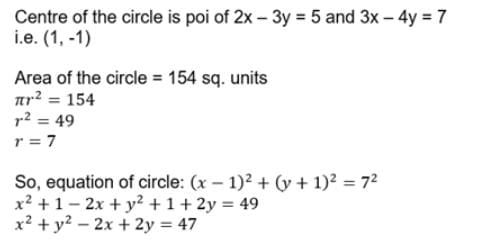QUESTION: 2

### If a be the radius of a circle which touches x-axis at the origin, then its equation is

Solution:

The equation of the circle  with centre at (h,k) and radius equal to a is (x−h)+(y−k)= a2
When the circle passes through the origin  and centre lies on x− axis
⇒h = a and k = 0
Then the equation (x−h)2+(y−k)2=abecomes (x−a)2+y2=a2
If a circle passes through the origin and centre lies on x−axis then the abscissa will be equal to the radius of the circle and the y−co-ordinate of the centre will be zero Hence, the equation of the circle will be of the form
(x±a)2+y2=a2⇒x2+a±2ax+y2=a2
=x2 +y±2ax=0 is the required equation of the circle.

QUESTION: 3

### The equation of the circle passing through (3, 6) and whose centre is (2, –1) is

Solution:
QUESTION: 4

The equation of a circle which passes through the three points (3, 0) (1, –6), (4, –1) is

Solution:

Gen equation of a circle is (x−h)2+(y−k)2=r2 (3,0),(1,−6),(4,−1) circle passes through these points
∴ These three points should satisfy the equation
of circle.
(3−h)2+k2=r2 ___ (I)
(1−h)2 +(−6−k)2 = r2(1−h)2+(6+k)2=r2  ___ (II)
From (I) & (II)
(3−h)2+k2 =(1−h)2+(6+k)2
9+h2−6h+k2 =1+h2 2h+36+k2+12k
9−6h=1−2h+36+12k
9−37=4h+12k
−28=4h+12k
h+3k=−7
h=−7−3k ___ (III)
(4−h)2+(−1−k)2=r2 ___ (IV)
From (I) & (IV)
(3−h)2+k2 = (4−h)2 +(1+k)2
9+h2 −6h+k2
=16+h2 −8h+1+k2+2k
9−6h=17−8h+2k
9−17=−2h+2k
−8=−2h+2k
−h+k=−4
k=−4+h ___ (V)
Put (V) in (III)
h=−7−3(−4+h)
h=−7+12−3h
h=5/4,k=−11/4,r=170/16
Eq of circle: (x−5/4)2 +(y+11/4)2 =170/16
x2+25/16−10x/4+y2 +121/16+22y/4=170/16
Simplifying, we get 2x2+xy2−5x+11y−3=0

QUESTION: 5

y = √3 + c1 & y = √3 + c2 are two parallel tangents of a circle of radius 2 units, then |c1 – c2| is equal to

Solution:

For both lines to be parallel tangent the distance between both lines
should be equal to the diameter of the circle
⇒ 4 = |c1−c2|/(1+3)1/2
⇒∣c1−c2∣ = 8

QUESTION: 6

B and C are fixed point having co-ordinates (3, 0) and (–3, 0) respectively. If the vertical angle BAC is 90º, then the locus of the centroid of the DABC has the equation

Solution:

Let A (a, b) and G (x. y) Now A, G, O are collinear
⇒ x = (2*0 + a)/3
⇒ a = 3x
and similarly b = 3y
Now (a,b) lies on the circle = 9x2 + 9y2 = 9
=> x2 + y2 = 1

QUESTION: 7

The area of an equilateral triangle inscribed in the circle x2 + y2 – 2x = 0 is

Solution: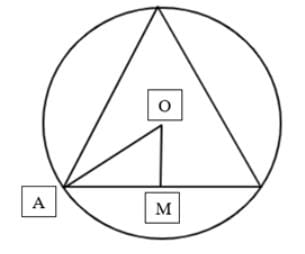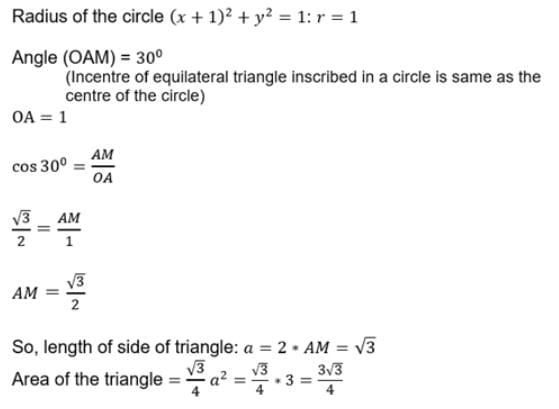QUESTION: 8

The length of intercept on y-axis, by a circle whose diameter is the line joining the points (–4,3) and (12,–1) is

Solution:

Equation of circle is given by,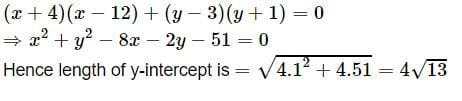QUESTION: 9

The gradient of the tangent line at the point (a cos a, a sin a) to the circle x2 + y2 = a2, is

Solution: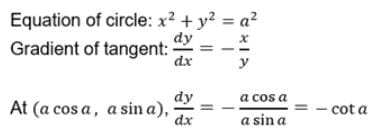QUESTION: 10

lx + my + n = 0 is a tangent line to the circle x2 + y2 = r2, if

Solution: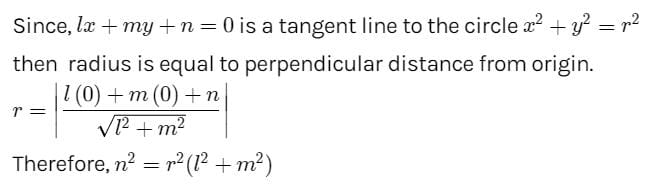QUESTION: 11

If y = c is a tangent to the circle x2+y2–2x+2y–2 = 0 at (1, 1), then the value of c is

Solution:

For line y=c to be tangent to the given circle at point (1,1)
It has to pass through (1,1)
⇒ c = 1

QUESTION: 12

Line 3x + 4y = 25 touches the circle x2 + y2 = 25 at the point

Solution:

Equation of tangent at (x1 , y1) to the circle x2 + y2 =25 is given by,
xx1 + yy1 = 25. Now comparing it with 3x+4y = 25
We get required point of contact (3,4)

QUESTION: 13

The equations of the tangents drawn from the point (0, 1) to the circle x2 + y2 - 2x + 4y = 0 are

Solution:

Let equation of tangent with slope =m and point (0,1)
(y−1)=m(x−0)⇒y=mx+1
Intersection point
x2+(mx+1)2−2x+4(mx+1)=0
(1+m2)x2+(−2+6m)x+5=0
For y=mx+1 to be tangent, discriminant =0
(6m−2)2−4×5(1+m2)=0
36m2+4−24m−20m2+20=0
16m2−20m+24=0
⇒ 2m2−3m−2=0
(2m+1)(m−2)=0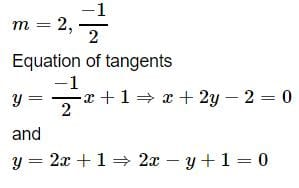QUESTION: 14

The greatest distance of the point P(10, 7) from the circle x2 + y2 – 4x – 2y – 20 = 0 is

Solution:
QUESTION: 15

The equation of the normal to the circle x2+y2 = 9 at the point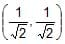is

Solution:

(x-x1)/(x1+g) = (y-y1)/(y1+g)
[x-1/(2)½]/(1/(2)½) = [y-1/(2)½]/(1/(2)½)
[(2x)½ -1 *(2)½]/(2)½ = [(2x)½ -1 *(2)½]/(2)½
(2x)½ -1 = (2y)½ -1
= x-y = 0

QUESTION: 16

The parametric coordinates of any point on the circle x2 + y2 – 4x – 4y = 0 are

Solution: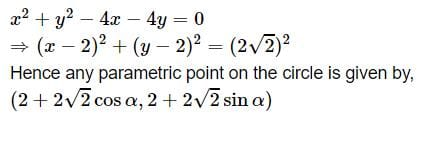QUESTION: 17

The length of the tangent drawn from the point (2, 3) to the circles 2(x2 + y2) – 7x + 9y – 11 = 0.

Solution: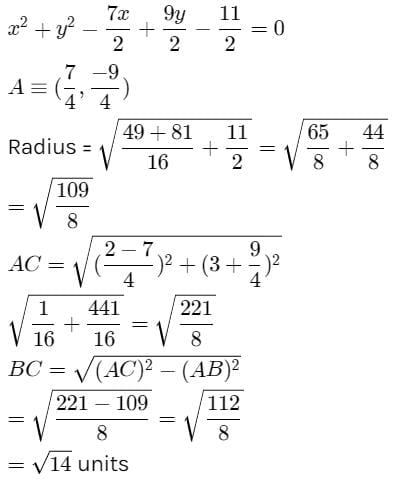QUESTION: 18

Tangents are drawn from (4, 4) to the circle x2 + y2 – 2x – 2y – 7 = 0 to meet the circle at A and B. The length of the chord AB is

Solution: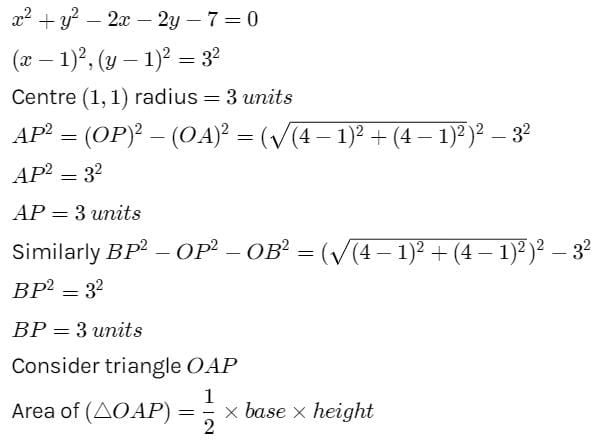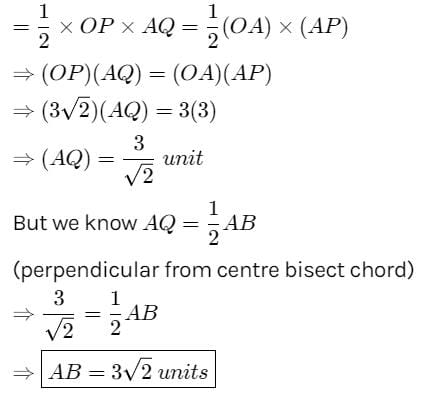QUESTION: 19

The angle between the two tangents from the origin to the circle (x – 7)2 + (y + 1)2 = 25 equals

Solution:

Let tangent from origin be y = mx
Using the condition of tangency, we get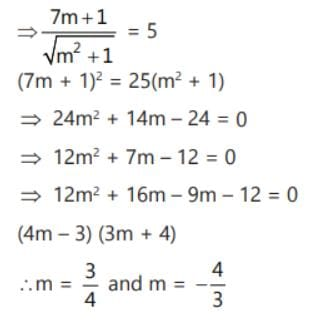The angle between tangents = π/2

QUESTION: 20

Pair of tangents are drawn from every point on the line 3x + 4y = 12 on the circle x2+ y2 = 4. Their variable chord of contact always passes through a fixed point whose co-ordinates are

Solution:

Let P(x1,y1) be a point on the line 3x + 4y = 12
Equation of variable chord of contact of P(x1,y1) w.r.t circle x2 + y2 = 4
xx1 + yy1 − 4 = 0   ...(1)
Also 3x1 + 4y1 − 12 = 0
⇒ x1 + 4/3y1 − 4 = 0   ...(2)
Comparing (1) and (2), we get
x = 1; y = 4/3
∴ Variable chord of contact always passes through (1, 4/3)

QUESTION: 21

The locus of the mid-points of the chords of the circle x2 + y2 – 2x – 4y – 11 = 0 which subtend 60º at the centre is

Solution:

Let AB be the chord of the circle and P be the midpoint of AB.
It is known that perpendicular from the center bisects a chord.
Thus △ACP is a right-angled triangle.
The equation of the give circle can be written as
(x−1)2+(y−2)2=16
Hence, centre C=(1,2) and radius =r=4 units.
PC=ACsin60degree
= rsin60degree
= 4([2(3)½]/2
= 2(3)1/2 units
Therefore, PC=2(3)1/2
⇒ PC2=12
⇒ (x−1)2+(y−2)2=12
⇒ x2+y2−2x−4y+5=12
⇒ x2+y2−2x−4y−7=0

QUESTION: 22

The locus of the centres of the circles such that the point (2, 3) is the mid point of the chord 5x + 2y = 16 is

Solution:

Slope of the given chord = −5/2
Slope of the line joining the midpoint on the chord and the centre of the circle = (3+f)/(2+g)
(5/2)[(3+f)/(2+g)] = −1
⇒ 15 + 5f = 4 + 2g
⇒ Locus is 2x − 5y + 11 = 0

QUESTION: 23

The equation of the circle having the lines y2 – 2y + 4x – 2xy = 0 as its normals & passing through the point (2, 1) is

Solution:

The normal line to circle is →y² - 2 y + 4 x -2 xy=0
→ y(y-2) - 2x(y-2)=0
→ (y-2)(y-2x)=0
the two lines are , y=2 and 2 x -y =0
The point of intersection of normals are centre of circle.
→ Put , y=2 in 2 x -y=0, we get
→2 x -2=0
→2 x=2
→ x=1
So, the point of intersection of normals is (1,2) which is the center of circle.
Also, the circle passes through (2,1).
Radius of circle is given by distance formula = [(1-2)² + (2-1)²]½
=(1+1)½ =(2)½
The equation of circle having center (1,2) and radius √2 is
= (x-1)²+(y-2)²=[√2]²
→ (x-1)²+(y-2)²= 2
x²+y² -2x-4y+3 = 0

QUESTION: 24

A circle is drawn touching the x-axis and centre at the point which is the reflection of (a, b) in the line y – x = 0. The equation of the circle is

Solution:

Radius of the circle = a
and centre ≡ (b,a)
And circle is touching the x-axis.
The equation of the circle :
x2+y2−2bx−2ay+b= 0

QUESTION: 25

The number of common tangents of the circles x2 + y2 – 2x – 1 = 0 and x2 + y2 – 2y – 7 = 0

Solution: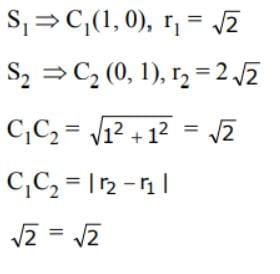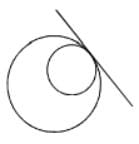Internally touch ∴ common tangent is one.

QUESTION: 26

The point from which the tangents to the circles x2 + y2 – 8x + 40 = 0,  5x2 + 5y2 – 25 x + 80 = 0, x2 + y2 – 8x + 16y + 160 = 0 are equal in length is

Solution:

The Required point is the radical centre of the three given circles. The radical axes of the three circles taken in pairs are 3x - 24 = 0,16y + 120 = 0 and - 3x + 16y + 80 = 0. On solving, the required point is (8, -15/2).

QUESTION: 27

If the circle x2 + y2 = 9 touches the circle x2 + y2 + 6y + c = 0, then c is equal to

Solution: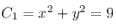touches the another circle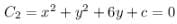Now, Central first circle will be
And its radius will be 3 units.
Also,centre of second circle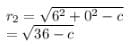As both touches each other
So,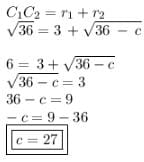QUESTION: 28

The tangent from the point of intersection of the lines 2x – 3y + 1 = 0 and 3x – 2y –1 = 0 to the circle x2 + y2 + 2x – 4y = 0 is

Solution: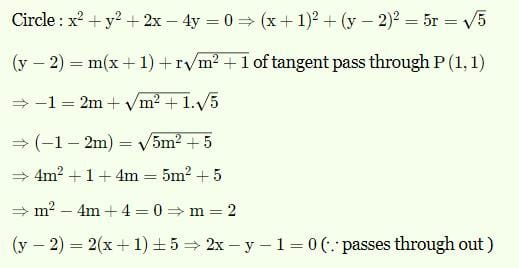QUESTION: 29

The length of the common chord of circles x2 + y2 – 6x – 16 = 0 and x2 + y2 – 8y – 9 = 0 is

Solution: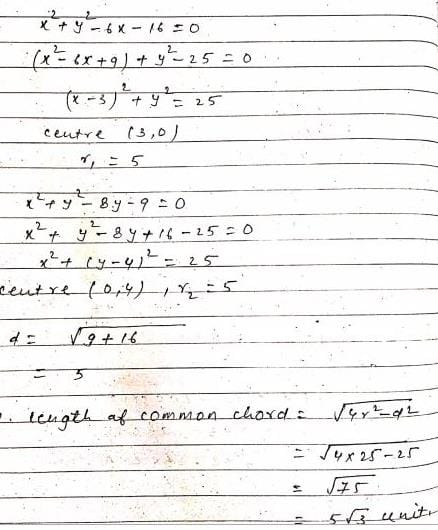QUESTION: 30

The distance between the chords of contact of tangents to the circle x2 + y2 + 2gx + 2fy + c = 0 from the origin and from the point (g, f) is

Solution: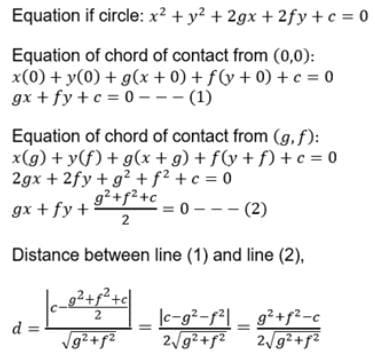Use Code STAYHOME200 and get INR 200 additional OFF Use Coupon Code